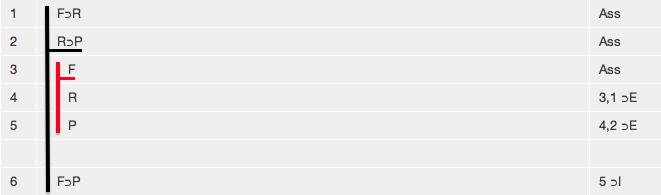# Review of Sentential Logic

Logical System

## Review of Sentential Logic

2013

You now have to tools to appraise sentential arguments.

Let us run through how these might be used with two examples.

#### Example 1.

Consider the argument

If no human action is free, then no one is responsible for what they do.
If no one is responsible for what they do, no one should be punished.
Therefore
If no human action is free, no one should be punished.

First it should be symbolized

F = no human action is free
R = no one is responsible for what they do
P = no one should be punished

F⊃R, R⊃P ∴ F⊃P

Then some thought should be given as to whether there is a counter-example and thus whether the argument is invalid. In a counter example the conclusion has to be false

 F ⊃ R R ⊃ P F ⊃ P F

but F⊃P is false only if F if true and P is false.

 F ⊃ R R ⊃ P F ⊃ P T F F

and these assignments also have to apply to the premises

 F ⊃ R R ⊃ P F ⊃ P T F T F F

but now we seem to be stuck for if R is true then the second premise is false but if R is false the first premise is false so it seems that we cannot find an assignment which renders all premises true and the conclusion false at one and the same time. It seems that this argument has no counter-example.

So now we try to give a derivation of it. And indeed we are able to findand this proves that the argument is valid.

#### Example 2.

Consider the argument

If the girl has spots in her mouth, then she has measles.
If the girl has spots on her back, then she has a heat rash.
The girl has spots on her back.
Therefore
The girl has a heat rash and does not have measles.

First it should be symbolized

S = the girl has spots in her mouth
M = the girl has measles
B = the girl has spots on her back
R = the girl has a heat rash

S⊃M, B⊃R, B ∴ R∧∼M

Then some thought should be given as to whether there is a counter-example and thus whether the argument is invalid. In a counter example all the premises have to be true and that tells us that B has to be assigned true

 S ⊃ M B ⊃ R B R & ∼ M T

and this assignment applies to all other B's

 S ⊃ M B ⊃ R B R & ~ M T T

the second premise B⊃R has to be true and given that B is true this means that R must be true (throughout the argument)

 S ⊃ M B ⊃ R B R & ∼ M T T T T T

the conclusion R∧M has to be false and given that R is true this must mean that ∼M is false and M true throughout the argument.

 S ⊃ M B ⊃ R B R & ∼ M T T T T T T F F T

all that remains is S and the first premise S⊃M-- M is true and we wish to have the whole premise true that means that S has can be either true or false but, just to pick one, we will assign it true

 S ⊃ M B ⊃ R B R & ∼ M T T T T T T T T F F T

This argument has a counter-example; the assignment B true, S true, M false and R true proves the argument to be invalid.